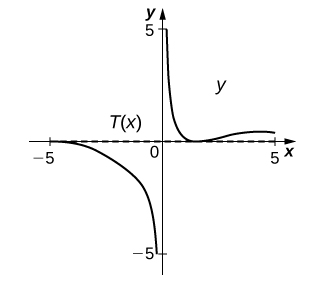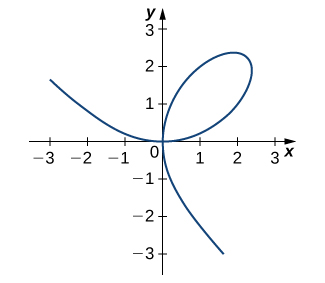$$\newcommand{\id}{\mathrm{id}}$$ $$\newcommand{\Span}{\mathrm{span}}$$ $$\newcommand{\kernel}{\mathrm{null}\,}$$ $$\newcommand{\range}{\mathrm{range}\,}$$ $$\newcommand{\RealPart}{\mathrm{Re}}$$ $$\newcommand{\ImaginaryPart}{\mathrm{Im}}$$ $$\newcommand{\Argument}{\mathrm{Arg}}$$ $$\newcommand{\norm}{\| #1 \|}$$ $$\newcommand{\inner}{\langle #1, #2 \rangle}$$ $$\newcommand{\Span}{\mathrm{span}}$$

# 3.8E: Exercises for Section 3.8

$$\newcommand{\vecs}{\overset { \rightharpoonup} {\mathbf{#1}} }$$ $$\newcommand{\vecd}{\overset{-\!-\!\rightharpoonup}{\vphantom{a}\smash {#1}}}$$$$\newcommand{\id}{\mathrm{id}}$$ $$\newcommand{\Span}{\mathrm{span}}$$ $$\newcommand{\kernel}{\mathrm{null}\,}$$ $$\newcommand{\range}{\mathrm{range}\,}$$ $$\newcommand{\RealPart}{\mathrm{Re}}$$ $$\newcommand{\ImaginaryPart}{\mathrm{Im}}$$ $$\newcommand{\Argument}{\mathrm{Arg}}$$ $$\newcommand{\norm}{\| #1 \|}$$ $$\newcommand{\inner}{\langle #1, #2 \rangle}$$ $$\newcommand{\Span}{\mathrm{span}}$$ $$\newcommand{\id}{\mathrm{id}}$$ $$\newcommand{\Span}{\mathrm{span}}$$ $$\newcommand{\kernel}{\mathrm{null}\,}$$ $$\newcommand{\range}{\mathrm{range}\,}$$ $$\newcommand{\RealPart}{\mathrm{Re}}$$ $$\newcommand{\ImaginaryPart}{\mathrm{Im}}$$ $$\newcommand{\Argument}{\mathrm{Arg}}$$ $$\newcommand{\norm}{\| #1 \|}$$ $$\newcommand{\inner}{\langle #1, #2 \rangle}$$ $$\newcommand{\Span}{\mathrm{span}}$$

In exercises 1 - 10, use implicit differentiation to find $$\dfrac{dy}{dx}$$.

1) $$x^2−y^2=4$$

2) $$6x^2+3y^2=12$$

$$\dfrac{dy}{dx}=\dfrac{−2x}{y}$$

3) $$x^2y=y−7$$

4) $$3x^3+9xy^2=5x^3$$

$$\dfrac{dy}{dx}=\dfrac{x}{3y}−\dfrac{y}{2x}$$

5) $$xy−\cos(xy)=1$$

6) $$y\sqrt{x+4}=xy+8$$

$$\dfrac{dy}{dx}=\dfrac{y−\dfrac{y}{2\sqrt{x+4}}}{\sqrt{x+4}−x}$$

7) $$−xy−2=\frac{x}{7}$$

8) $$y\sin(xy)=y^2+2$$

$$\dfrac{dy}{dx}=\dfrac{y^2\cos(xy)}{2y−\sin(xy)−xy\cos(xy)}$$

9) $$(xy)^2+3x=y^2$$

10) $$x^3y+xy^3=−8$$

$$\dfrac{dy}{dx}=\dfrac{−3x^2y−y^3}{x^3+3xy^2}$$

For exercises 11 - 16, find the equation of the tangent line to the graph of the given equation at the indicated point. Use a calculator or computer software to graph the function and the tangent line.

11) [T] $$x^4y−xy^3=−2, \quad (−1,−1)$$

12) [T] $$x^2y^2+5xy=14,\quad (2,1)$$

$$y=−\frac{1}{2}x+2$$13) [T] $$\tan(xy)=y,\quad \left(\frac{π}{4},1\right)$$

14) [T] $$xy^2+\sin(πy)−2x^2=10, \quad (2,−3)$$

$$y=\frac{1}{π+12}x−\frac{3π+38}{π+12}$$15) [T] $$\dfrac{x}{y}+5x−7=−\frac{3}{4}y, \quad (1,2)$$

16) [T] $$xy+\sin(x)=1,\quad \left(\frac{π}{2},0\right)$$

$$y=0$$17) [T] The graph of a folium of Descartes with equation $$2x^3+2y^3−9xy=0$$ is given in the following graph.a. Find the equation of the tangent line at the point $$(2,1)$$. Graph the tangent line along with the folium.

b. Find the equation of the normal line to the tangent line in a. at the point $$(2,1)$$.

18) For the equation $$x^2+2xy−3y^2=0,$$

a. Find the equation of the normal to the tangent line at the point $$(1,1)$$.

b. At what other point does the normal line in a. intersect the graph of the equation?

a. $$y=−x+2$$
b. $$(3,−1)$$

19) Find all points on the graph of $$y^3−27y=x^2−90$$ at which the tangent line is vertical.

20) For the equation $$x^2+xy+y^2=7$$,

a. Find the $$x$$-intercept(s).

b.Find the slope of the tangent line(s) at the $$x$$-intercept(s).

c. What does the value(s) in part b. indicate about the tangent line(s)?

a. $$\left(±\sqrt{7},0\right)$$
b. $$−2$$
c. They are parallel since the slope is the same at both intercepts.

21) Find the equation of the tangent line to the graph of the equation $$\sin^{−1}x+\sin^{−1}y=\frac{π}{6}$$ at the point $$\left(0,\frac{1}{2}\right)$$.

22) Find the equation of the tangent line to the graph of the equation $$\tan^{−1}(x+y)=x^2+\frac{π}{4}$$ at the point $$(0,1)$$.

$$y=−x+1$$

23) Find $$y′$$ and $$y''$$ for $$x^2+6xy−2y^2=3$$.

24) [T] The number of cell phones produced when $$x$$ dollars is spent on labor and $$y$$ dollars is spent on capital invested by a manufacturer can be modeled by the equation $$60x^{3/4}y^{1/4}=3240$$.

a. Find $$\frac{dy}{dx}$$ and evaluate at the point $$(81,16)$$.

b. Interpret the result of a.

a. $$\frac{dy}{dx}=−0.5926$$
b. When $81 is spent on labor and$16 is spent on capital, the amount spent on capital is decreasing by $0.5926 per$1 spent on labor.

25) [T] The number of cars produced when $$x$$ dollars is spent on labor and $$y$$ dollars is spent on capital invested by a manufacturer can be modeled by the equation $$30x^{1/3}y^{2/3}=360$$.

(Both $$x$$and $$y$$ are measured in thousands of dollars.)

a. Find $$\frac{dy}{dx}$$ and evaluate at the point $$(27,8)$$.

b. Interpret the result of part a.

26) The volume of a right circular cone of radius $$x$$ and height $$y$$ is given by $$V=\frac{1}{3}πx^2y$$. Suppose that the volume of the cone is $$85π\,\text{cm}^3$$. Find $$\dfrac{dy}{dx}$$ when $$x=4$$ and $$y=16$$.

$$\dfrac{dy}{dx} = −8$$

For exercises 27 - 28, consider a closed rectangular box with a square base with side $$x$$ and height $$y$$.

27) Find an equation for the surface area of the rectangular box, $$S(x,y)$$.

28) If the surface area of the rectangular box is 78 square feet, find $$\dfrac{dy}{dx}$$ when $$x=3$$ feet and $$y=5$$ feet.

$$\dfrac{dy}{dx} = −2.67$$

In exercises 29 - 31, use implicit differentiation to determine $$y′$$. Does the answer agree with the formulas we have previously determined?

29) $$x=\sin y$$

30) $$x=\cos y$$

$$y′=−\dfrac{1}{\sqrt{1−x^2}}$$
31) $$x=\tan y$$